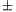Mathematical and Physical Journal
for High Schools
Issued by the MATFUND Foundation
 Already signed up? New to KöMaL?

# KöMaL Problems in Mathematics, April 2013

Show/hide problems of signs:## Problems with sign 'C'

Deadline expired on May 10, 2013.

C. 1165. How many seven-digit natural numbers are there in which the digits, read from left to right, form a nondecreasing sequence (e.g. 2444689)?

(5 pont)

solution (in Hungarian), statistics

C. 1166. A prime number p is four greater than the square of an integer n and the double of p is one less than the cube of n. Find p.

(5 pont)

solution (in Hungarian), statistics

C. 1167. The line segments AA1, BB1, CC1 are the angle bisectors of a triangle ABC. Through the endpoint of the bisector of each angle, draw parallels to the lines forming the angle. Consider the segments of these six lines that lie in the interior of the triangle. Prove that the sum of their lengths cannot be longer than the perimeter of the triangle.

(5 pont)

solution (in Hungarian), statistics

C. 1168. Prove that.

(5 pont)

solution (in Hungarian), statistics

C. 1169. Consider a sphere of diameter d, a cylinder whose diameter and height are both d, and a cone of base diameter d. What may be the height of the cone if the volumes of the three solids form an arithmetic progression in some order?

(5 pont)

solution (in Hungarian), statistics## Problems with sign 'B'

Deadline expired on May 10, 2013.

B. 4532. In a given n×n table, n-1 fields are marked. A move consists in interchanging two rows or two columns. Is it always possible to carry out an appropriate sequence of such moves, so that all marked fields terminate under the main diagonal? (The main diagonal of a table connects the top left and bottom right corners.)

(Matlap, Kolozsvár)

(4 pont)

solution (in Hungarian), statistics

B. 4533. Is there a positive integer that is a perfect power and has digits of 0 and 6 only in decimal notation?

(4 pont)

solution (in Hungarian), statistics

B. 4534. M and N are points on the longest side, AB of a triangle ABC, such that BM=BC and AN=AC. The parallel drawn through point M to the side BC intersects side AC at P, and the parallel drawn through point N to the side AC intersects side BC at Q. Prove that CP=CQ.

(Kvant)

(3 pont)

solution (in Hungarian), statistics

B. 4535. Solve the equation (x2-x-1)2-x3=5.

(5 pont)

solution (in Hungarian), statistics

B. 4536. Point P lies in the plane of a triangle ABC. The orthogonal projections of P onto the altitudes of the triangle are A1, B1 and C1. Given that AA1=BB1=CC1, determine the point P, and find the equal length of these three line segments.

(5 pont)

solution (in Hungarian), statistics

B. 4537. 200 line segments of unit length are placed in a 20×20 square. Prove that there exists a disc of unit diameter in the square such that it does not have a point in common with any of the line segments.

(Transcarpathian competition problem)

(5 pont)

solution (in Hungarian), statistics

B. 4538. The functionsatisfies (x-2)f(x)-(x+1)f(x-1)=3. Evaluate f(2013), given that f(2)=5.

(Matlap, Kolozsvár)

(5 pont)

solution (in Hungarian), statistics

B. 4539. The centroid of a triangle ABC is S, and its circumcentre is K. The centres of the circumscribed circles of the triangles BCS, CAS and ABS are P, Q and R. Prove that K is the centroid of triangle PQR.

(Suggested by Zs. Sárosdi, Veresegyház)

(5 pont)

solution (in Hungarian), statistics

B. 4540. There are n prisoners in a prison. The guards were bored, so they invented the following game: In the courtyard, they place either a red cap or a blue cap on the head of each prisoner. The prisoners are then given some time to look at one another (each prisoner can see all caps but his own), and then each of them has to write down on a sheet of paper what colour he thinks his cap is. Those guessing correctly are allowed to walk in the courtyard the following day, too. What is the largest number k for which the prisoners may follow an appropriate strategy to make it certain that at least k of them are allowed to walk in the courtyard the following day?

(6 pont)

solution (in Hungarian), statistics

B. 4541. Letbe the convex hull of the points with coordinates (1;1;1), (2;4;8), ..., (100;1002;1003). How many vertices, edges and faces doeshave?

(6 pont)

solution (in Hungarian), statistics## Problems with sign 'A'

Deadline expired on May 10, 2013.

A. 587. Find all positive integers n for which there exists a sequence a1<a2<...<an of positive integers with the property that the sums ai+aj with 1i<jn are distinct and among them each modulo 4 residue occurs the same number of times.

(Proposed by Ilya Bogdanov, Moscow)

(5 pont)

statistics

A. 588. Let a be a positive integer. Prove that all prime divisors of 7a2(a+1)-1 are of the form 7k1.

(5 pont)

solution (in Hungarian), statistics

A. 589. Does there exist such a convex polyhedron that can be placed in a sphere with unit radius, it has at least 2013 vertices, it has no edge shorter than 1/2, and it has no triangle face?

(5 pont)

solution (in Hungarian), statistics